# Recursion Continued Tail Recursion versus Iterative Looping Using

• Slides: 26Recursion (Continued) • Tail Recursion versus Iterative Looping • Using Recursion – Printing numbers in any base – Computing Greatest Common Denominator – Towers of Hanoi • Analyzing Recursive Algorithms • Misusing Recursion – Computing Fibonacci numbers • The Four Fundamental Rules of Recursion • Reading L&C 7. 3 – 7. 4 1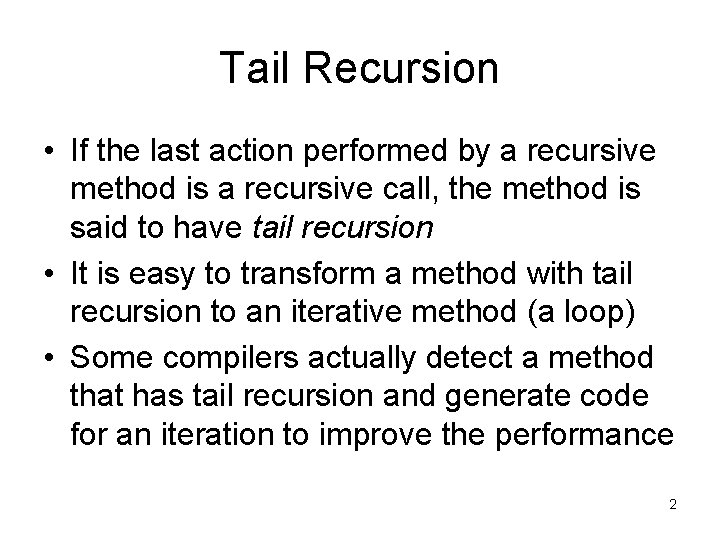Tail Recursion • If the last action performed by a recursive method is a recursive call, the method is said to have tail recursion • It is easy to transform a method with tail recursion to an iterative method (a loop) • Some compilers actually detect a method that has tail recursion and generate code for an iteration to improve the performance 2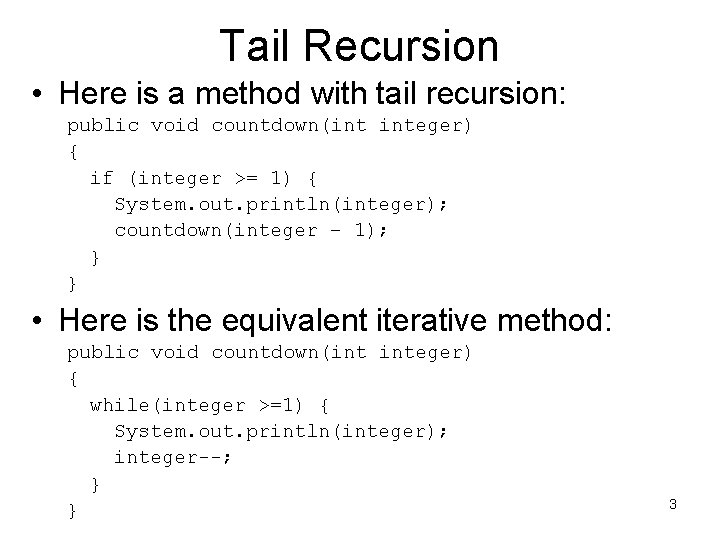Tail Recursion • Here is a method with tail recursion: public void countdown(int integer) { if (integer >= 1) { System. out. println(integer); countdown(integer – 1); } } • Here is the equivalent iterative method: public void countdown(int integer) { while(integer >=1) { System. out. println(integer); integer--; } } 3Tail Recursion • As you can see, conversion from tail recursion to a loop is straightforward • Change the if statement that detects the base case to a while statement with the same condition • Change recursive call with a modified parameter value to a statement that just modifies the parameter value • Leave the rest of the body the same 4Tail Recursion • Let’s look at the factorial method again • Does it have tail recursion? private int factorial(int n) { return n == 1? 1 : n * factorial(n – 1); } • Although the recursive call is in the last line of the method, the last operation is the multiplication of the return value by n • Therefore, this is not tail recursion 5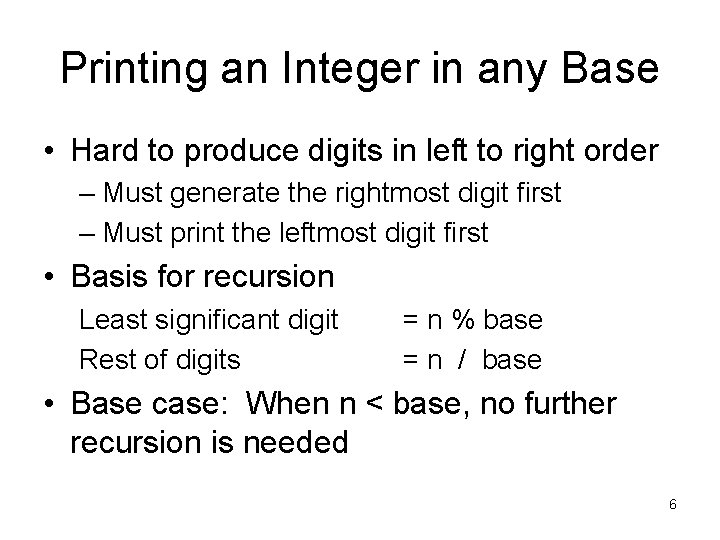Printing an Integer in any Base • Hard to produce digits in left to right order – Must generate the rightmost digit first – Must print the leftmost digit first • Basis for recursion Least significant digit Rest of digits = n % base = n / base • Base case: When n < base, no further recursion is needed 6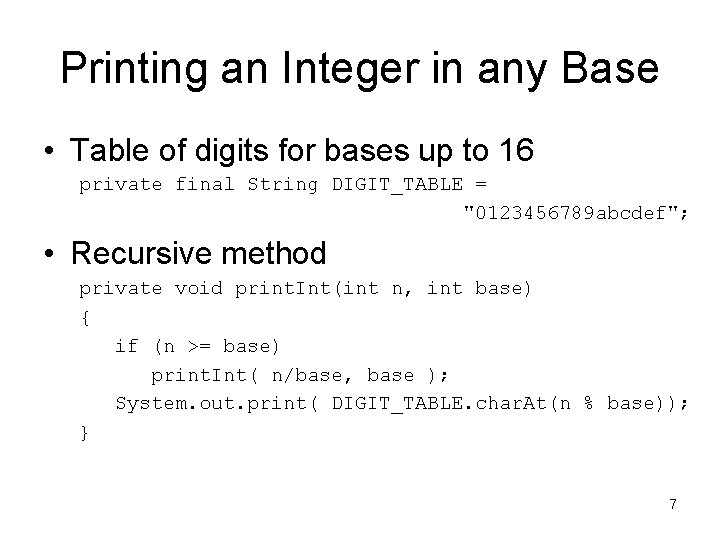Printing an Integer in any Base • Table of digits for bases up to 16 private final String DIGIT_TABLE = "0123456789 abcdef"; • Recursive method private void print. Int(int n, int base) { if (n >= base) print. Int( n/base, base ); System. out. print( DIGIT_TABLE. char. At(n % base)); } 7Computing GCD of A and B • Basis for recursion GCD (a, 0) = a GCD (a, b) = GCD (b, a mod b) (base case) (recursion) • Recursive method private int gcd(int a, int b) { if (b != 0) return gcd(b, a % b); return a; } // recursion // base case 8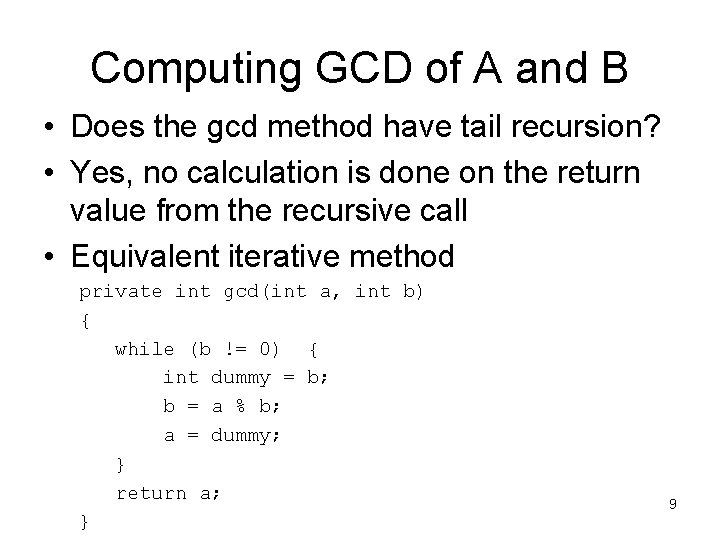Computing GCD of A and B • Does the gcd method have tail recursion? • Yes, no calculation is done on the return value from the recursive call • Equivalent iterative method private int gcd(int a, int b) { while (b != 0) { int dummy = b; b = a % b; a = dummy; } return a; } 9Towers of Hanoi • The Towers of Hanoi puzzle was invented by a French mathematician, Edouard Lucas in 1883. (See “Ancient Folklore”) • There are three upright pegs and a set of disks with holes to fit over the pegs • Each disk has a different diameter and a disk can only be put on top of a larger disk • Must move a pile of N disks from a starting tower to an ending tower one at a time 10Towers of Hanoi • • This bit of ancient folklore was invented by De Parville in 1884. ``In the great temple at Benares, says he, beneath the dome which marks the centre of the world, rests a brass plate in which are fixed three diamond needles, each a cubit high and as thick as the body of a bee. On one of these needles, at the creation, God placed sixty-four discs of pure gold, the largest disc resting on the brass plate, and the others getting smaller and smaller up to the top one. This is the Tower of Bramah. Day and night unceasingly the priests transfer the discs from one diamond needle to another according to the fixed and immutable laws of Bramah, which require that the priest on duty must not move more than one disc at a time and that he must place this disc on a needle so that there is no smaller disc below it. When the sixty-four discs shall have been thus transferred from the needle on which at the creation God placed them to one of the other needles, tower, temple, and Brahmins alike will crumble into dust, and with a thunderclap the world will vanish. '' (W W R Ball, MATHEMATICAL RECREATIONS AND ESSAYS, p. 304) 11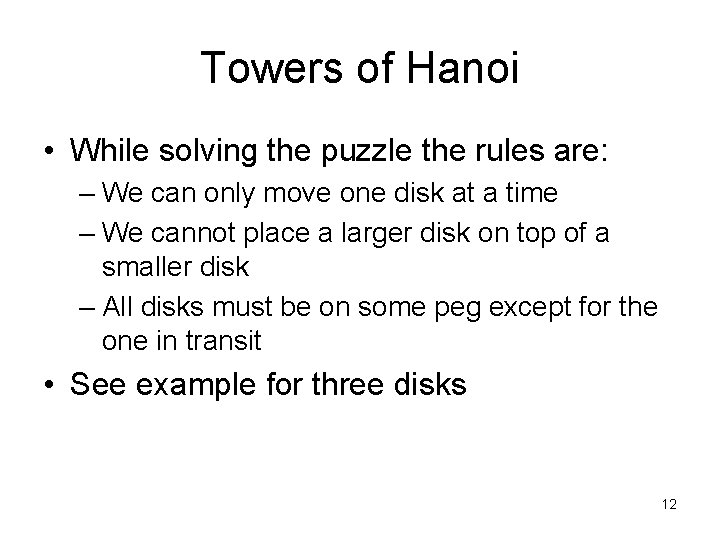Towers of Hanoi • While solving the puzzle the rules are: – We can only move one disk at a time – We cannot place a larger disk on top of a smaller disk – All disks must be on some peg except for the one in transit • See example for three disks 12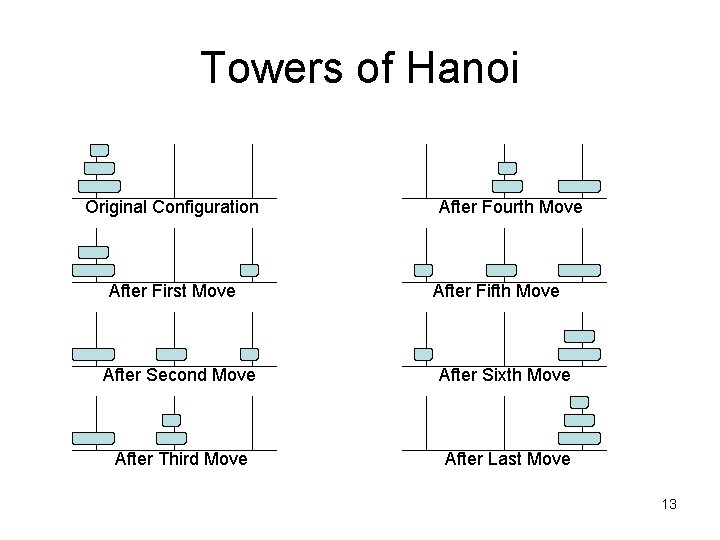Towers of Hanoi Original Configuration After First Move After Fourth Move After Fifth Move After Second Move After Sixth Move After Third Move After Last Move 13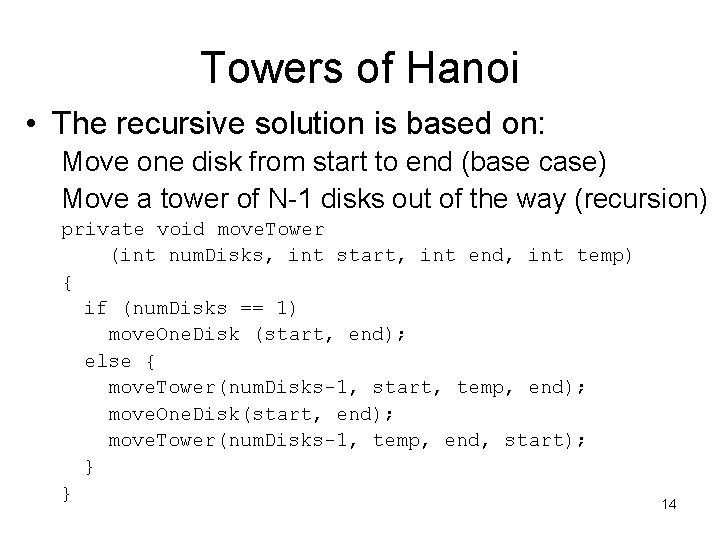Towers of Hanoi • The recursive solution is based on: Move one disk from start to end (base case) Move a tower of N-1 disks out of the way (recursion) private void move. Tower (int num. Disks, int start, int end, int temp) { if (num. Disks == 1) move. One. Disk (start, end); else { move. Tower(num. Disks-1, start, temp, end); move. One. Disk(start, end); move. Tower(num. Disks-1, temp, end, start); } } 14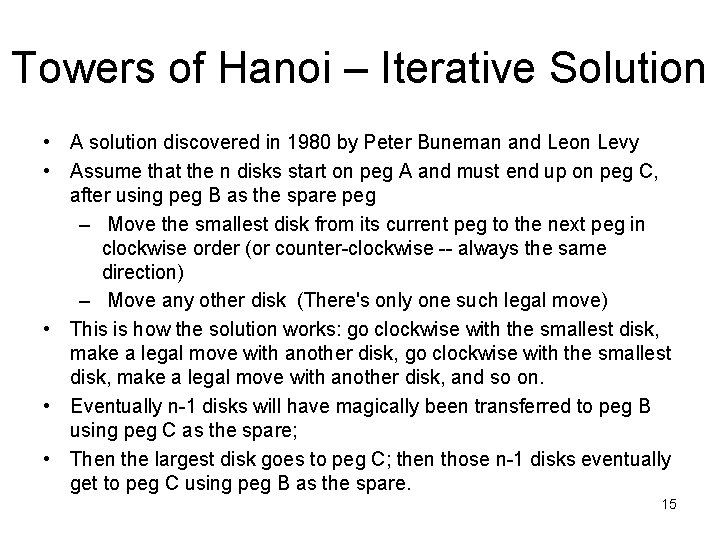Towers of Hanoi – Iterative Solution • A solution discovered in 1980 by Peter Buneman and Leon Levy • Assume that the n disks start on peg A and must end up on peg C, after using peg B as the spare peg – Move the smallest disk from its current peg to the next peg in clockwise order (or counter-clockwise -- always the same direction) – Move any other disk (There's only one such legal move) • This is how the solution works: go clockwise with the smallest disk, make a legal move with another disk, and so on. • Eventually n-1 disks will have magically been transferred to peg B using peg C as the spare; • Then the largest disk goes to peg C; then those n-1 disks eventually get to peg C using peg B as the spare. 15Analyzing Recursive Algorithms • To determine the order of a recursive algorithm: – Determine the order of the recursion (the number of times the recursive definition is followed) – Multiply by the order of the body • For n!, the order of the recursion is O(n) and the order of the body is O(1) - a single multiplication - so the algorithm is O(n) 16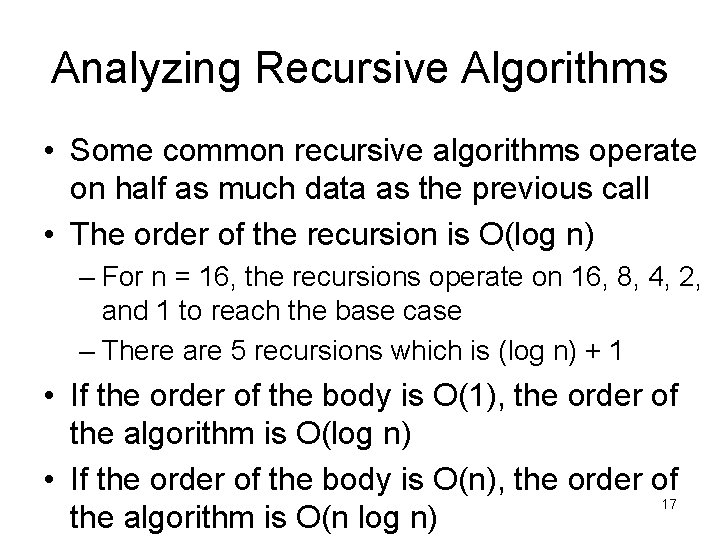Analyzing Recursive Algorithms • Some common recursive algorithms operate on half as much data as the previous call • The order of the recursion is O(log n) – For n = 16, the recursions operate on 16, 8, 4, 2, and 1 to reach the base case – There are 5 recursions which is (log n) + 1 • If the order of the body is O(1), the order of the algorithm is O(log n) • If the order of the body is O(n), the order of 17 the algorithm is O(n log n)Analyzing Recursive Algorithms • The towers of Hanoi algorithm is analyzed based on the number of disks to be moved – Each call to move. Tower results in one disk being moved – However, each call results in two recursive calls to move. Tower – Each recursion operates on only 1 less disk than the number of disks for the previous call • Hence the order is O(2 n) 18Analyzing Recursive Algorithms • For the towers of Hanoi with 64 disks and one move being made every second, the solution will take over 584 billion years • However, this is not the fault of a recursive algorithm being used • The problem itself is that time consuming regardless of how it is solved • Misusing recursion means that a recursive solution is unnecessarily worse than a loop 19Misusing Recursion • Some algorithms are stated in a recursive manner, but they are not good candidates for implementation as a recursive program • Calculation of the sequence of Fibonacci numbers Fn (which have many interesting mathematical properties) can be stated as: F 0 = 0 F 1 = 1 Fn = F(n-1) + F (n-2) (one base case) (another base case) (the recursion) 20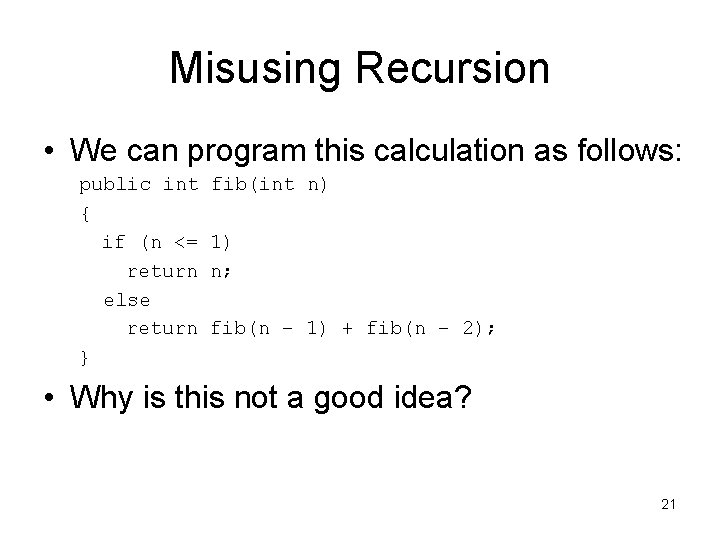Misusing Recursion • We can program this calculation as follows: public int { if (n <= return else return } fib(int n) 1) n; fib(n – 1) + fib(n – 2); • Why is this not a good idea? 21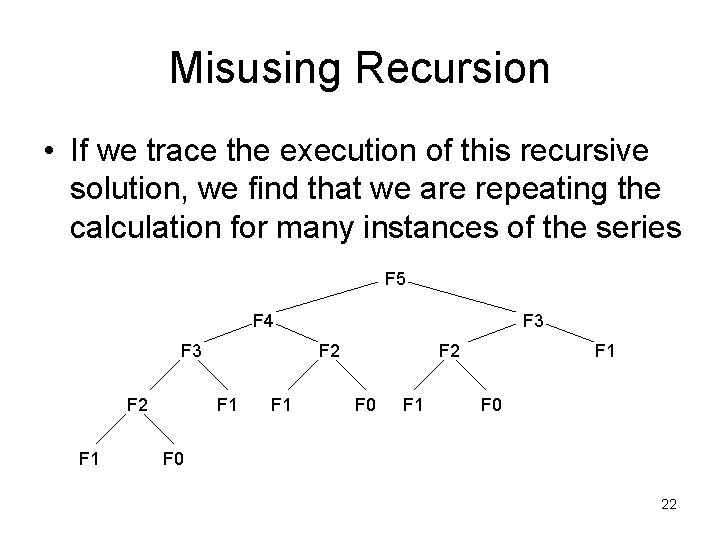Misusing Recursion • If we trace the execution of this recursive solution, we find that we are repeating the calculation for many instances of the series F 5 F 4 F 3 F 2 F 1 F 1 F 2 F 0 F 1 F 0 22Misusing Recursion • Note that in calculating F 5, our code is calculating F 3 twice, F 2 three times, F 1 five times, and F 0 three times • These duplicate calculations get worse as the number N increases • The order of the increase of time required with the value of N is exponential O(2 N) • For N = 40, the total number of recursive calls is more than 300, 000 23Misusing Recursion • This iterative solution (for N >= 2) uses a form of “dynamic programming” and is O(n) : public int fibonacci(int n) { int f. N = 0; int f. Nminus 2 = 0, f. Nminus 1 = 1; for (int i = 2; i <= n; i++) { f. N = f. Nminus 1 + f. Nminus 2; f. Nminus 2 = f. Nminus 1; f. Nminus 1 = f. N; } return f. N; 24 }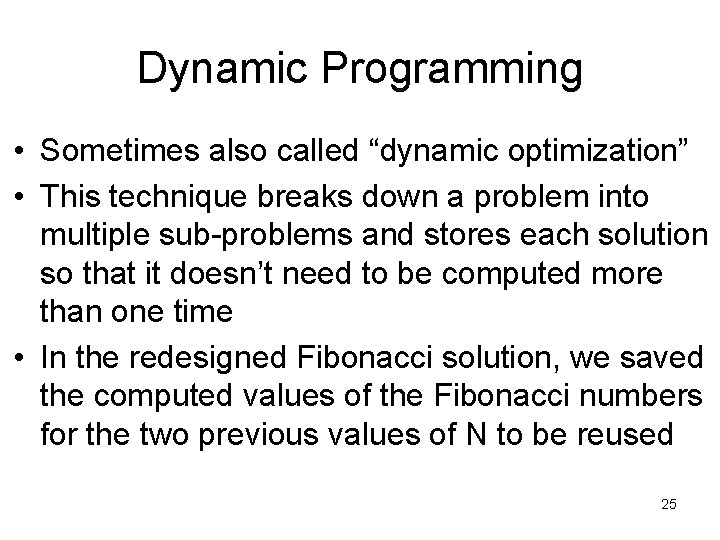Dynamic Programming • Sometimes also called “dynamic optimization” • This technique breaks down a problem into multiple sub-problems and stores each solution so that it doesn’t need to be computed more than one time • In the redesigned Fibonacci solution, we saved the computed values of the Fibonacci numbers for the two previous values of N to be reused 25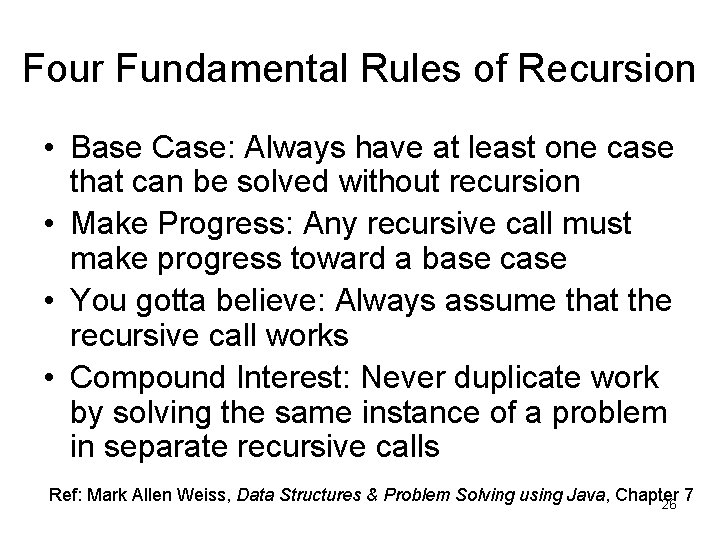Four Fundamental Rules of Recursion • Base Case: Always have at least one case that can be solved without recursion • Make Progress: Any recursive call must make progress toward a base case • You gotta believe: Always assume that the recursive call works • Compound Interest: Never duplicate work by solving the same instance of a problem in separate recursive calls Ref: Mark Allen Weiss, Data Structures & Problem Solving using Java, Chapter 7 26